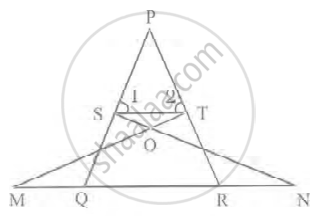# In figure, if ∠1 =∠2 and ΔNSQ ≅ ΔMTR, then prove that ΔPTS ~ ΔPRQ. - Mathematics

Sum

In figure, if ∠1 =∠2 and ΔNSQ ≅ ΔMTR, then prove that ΔPTS ~ ΔPRQ.#### Solution

ΔNSQ ≅ ΔMTR

∠1 = ∠2

Since,

∆NSQ = ∆MTR

So,

SQ = TR  ......(i)

Also,

∠1 = ∠2 ⇒ PT = PS  ......(ii)

Since, sides opposite to equal angles are also equal

From Equation (i) and (ii).

(PS)/(SQ) = (PT)/(TR)

⇒ ST || QR

By converse of basic proportionality theorem, If a line is drawn parallel to one side of a triangle to intersect the other sides in distinct points, the other two sides are divided in the same ratio.

∴ ∠1 = PQR

And

∠2 = ∠PRQ

In ∆PTS and ∆PRQ.

∠P = ∠P   ......[Common angles]

∠1 = ∠PQR  .....(Proved)

∠2 = ∠PRQ   ....(Proved)

∴ ∆PTS – ∆PRQ   ......[By AAA similarity criteria]

Hence proved.

Concept: Basic Proportionality Theorem (Thales Theorem)
Is there an error in this question or solution?

#### APPEARS IN

NCERT Mathematics Exemplar Class 10
Chapter 6 Triangles
Exercise 6.3 | Q 3 | Page 67

Share